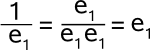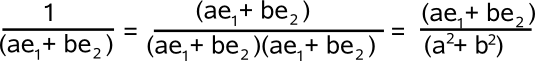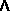# Maths - Multivector Functions

We will derive multivector equivalents to the quaternion and complex number idea of the conjugate, so lets start by reviewing that concept:

## Comparison to Quaternions

On the Quaternion and Complex number pages (here) we described the conjugate. It is a quaternion with the same magnitudes but with the sign of the imaginary parts changed, so:

• conj(a + b i + c j + d k) = a - b i - c j - d k

The notation for the conjugate of a quaternion 'q' is either of the following:

• conj(q)
• q'

The conjugate is useful because it has the following properties:

• qa' * qb' = (qb*qa)' In this way we can change the order of the multiplicands.
• q * q' = a2 + b2 + c2 + d2 = real number. Multiplying a quaternion by its conjugate gives a real number. This makes the conjugate useful for finding the multiplicative inverse. For instance, if we are using a quaternion q to represent a rotation then conj(q) represents the same rotation in the reverse direction.
• Pout = q * Pin * q' We use this to calculate a rotation transform.

It would be useful to be able to do similar things with multivectors and so we investigate the following functions:

## Inverse

To investigate how to calculate the multiplicative inverse of multivector 'm', that is '1/m' then we will start from the simplest case and gradually work up to more complex cases.

In standard vector algebra there is not an inverse, in geometric algebra vectors do have an inverse, but not all multivectors have an inverse, as we shall see later.

So, first we will take the inverse of a base vector 1/e1,We multiply top and bottom by the same vector, since the square of the vector is a scalar value, the bottom part cancels out and the vector is its own inverse.

We can also do the same when the vector is the linear combination of vector bases.So again the vector is its own inverse, with a scaling factor being the division by the scalar (a² + b²).

So lets move on to pure bivectors. We can invert a basis bivector by multiplying the top & bottom by its reverse, in other words we reverse the order of its terms, the reverse function is discussed below.This reversal makes it very easy to cancel out the denominator as like terms are adjacent and as the middle term cancels out the outer terms come together and can be cancelled out. For the rules used when calculating the results of products see this page. (adjacent like term cancel out and reversing the order changes the sign)

We can also calculate the inverse of a linear combination of bivector bases as follows.So to take the inverse we can separately take the reverse of each term (and apply the scaling factor).

There is a problem though, the non scalar terms only cancel out because e12 and e23 have a common term, otherwise there will be a non scalar term in the denominator. In the case of 3 dimensional vectors this is not a problem, because there will always be a common term, but four dimensional bivectors don't in general have a multiplicative inverse.

So what about higher order terms and multivectors containing a mixture of different blades?

## Reverse

The reverse function of a multivector reverses the order of its factors, including the order of the base values within a component. The reverse function is denoted by †, so the reversal of A is denoted by A†.

so for 3D vectors, if A = B† then:

a.e = b.e321 = -b.e123
a.e1 = b.e32 = -b.e23
a.e2 = b.e13 = -b.e31
a.e3 = b.e21 = -b.e12
a.e12 = b.e3
a.e31 = b.e2
a.e23 = b.e1
a.e123 = b.e

The reversal function is important for a number of reasons, one reason is that it can map a multiplication into another multiplication with the order of the multiplicands reversed:

(A * B)† = B†* A†

We can think of this as a morphism where † maps to an equivalent expression with order of multiplication reversed.Another application for the reversal function is to specify a transformation from one vector field to another:

pout = A pin A†

In other words, if pin is a pure vector (i.e. real, bivector and tri-vector parts are all zero) then pout will also be a pure vector. I would appreciate any proof of this.If you have a proof, that I could add to this page, please let me know, In the 3D case I guess I could try the brute force approach of multiplying out the terms for a general expression A and p.

I think this may also apply to:

pout = A pin A -1

but not every multivector is invertible, one condition that should ensure that a multivector is invertible is:

A A† = 1

## Dual

The dual of a grade-r multivector Ar is the product of Arwith the grade-n pseudoscalar I. This has grade n-r.

Ar* = dual of Ar which has grade n-r.

Also known as Hodge Dual

Let I = the pseudoscalar

And if this is normalised:

|I2| = 1

The textbooks (such as Hestenes) choose 'I' such that it has the orientation specified by a right-handed set of vectors in 3D, the rest of this site uses a left hand coordinate system, so what should we use?

I is known as the dextral unit psudoscalar

Identities

Ar* = I Ar = (-1)r(n-1)Ar I

Ar • (Bs I) = (ArBs) I

where:

• Ar = blade r of multivector A
• n = grade of multivector
• I = the pseudoscalar## Conjugate

This reverses all directions in space

A~ denotes the conjugate of A

conjugate, reverse and dual are related as follows.

A~= (A†)* = (A*)†

identities

(A * B)~ = B~* A~

## Norm

under construction

## Exponential

We define the exponential of multivector a as ea º åi=0¥ ai / i ! .
In particular:
ea is the traditional scalar exponential function
For any pure square multivector [ a2 = ±|a|2 ] we have ea = cos|a| + a~ sin|a| if a2 < 0 ;
cosh|a| + a~ sinh|a| if a2 > 0 ;
1+a if a2 = 0.

For unit multivector a:
eafeay = ea(f+y)
(d/df) elaf =lea(lf+p/2) =laelaf

## Involution

a# = sum k=0(N (-1)k a <k> = a<+> - a<-> .

## Determinant

The determinant of a multivector can be calculated from the table shown for the multipication of each dimentional vectors.

Book Shop - Further reading.

Where I can, I have put links to Amazon for books that are relevant to the subject, click on the appropriate country flag to get more details of the book or to buy it from them.Clifford Algebra to Geometric Calculus: A Unified Language for Mathematics and Physics (Fundamental Theories of Physics). This book is intended for mathematicians and physicists rather than programmers, it is very theoretical. It covers the algebra and calculus of multivectors of any dimension and is not specific to 3D modelling.